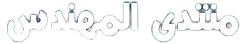# Earned Value Excel Template

## Overview:

This excel sheet is useful for earned value calculation in any construction project.

## Planned Value:

PV = Percent Complete (planned) x Task Budget

## Earned Value:

EV = Percent Complete (actual) x Task Budget

## Actual Cost:

AC = Actual Cost of the Task

SV = EV – PV

## Schedule Performance Index:

SPI = EV/PV

• If SPI < 1, the task is behind schedule
• If SPI = 1, the task is on schedule

## Cost Variance:

CV = EV – AC

• If CV is negative, the task is over budget
• If CV is zero, the project is on budget
• If CV is positive, the project is under budget

## Cost Performance Index:

CPI = EV/AC

• If CPI < 1, the task is over budget
• If CPI = 1, the task is on budget
• If CPI > 1, the task is under budget

## Budget At Completion:

BAC = Project Budget

## Estimate At Completion:

EAC = BAC/CPI

EAC = AC + (BAC – EV)

EAC = AC + [(BAC – EV)/(SPI x CPI)]

EAC = AC + ETC

ETC = EAC – AC

VAC = BAC – EAC

## To Complete Performance Index:

TCPI = (BAC – EV) / (BAC – AC)

TCPI = (BAC – EV) / (EAC – AC)

التحميل
EVM Excel.zip (645.0% u)

المصدر